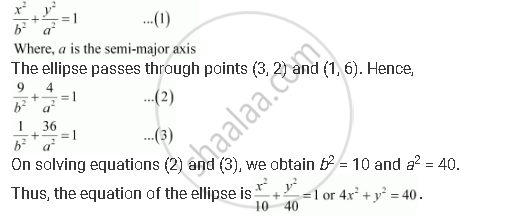CBSE (Arts) Class 11CBSE
Share
Notifications

View all notifications
Books Shortlist
Your shortlist is empty

# Find the Equation for the Ellipse that Satisfies the Given Conditions: Centre at (0, 0), Major Axis on the Y-axis and Passes Through the Points (3, 2) and (1, 6) - CBSE (Arts) Class 11 - Mathematics

Login
Create free account

Forgot password?
ConceptEllipse Standard Equations of an Ellipse

#### Question

Find the equation for the ellipse that satisfies the given conditions: Centre at (0, 0), major axis on the y-axis and passes through the points (3, 2) and (1, 6)

#### Solution

Since the centre is at (0, 0) and the major axis is on the y-axis, the equation of the ellipse will be of the formIs there an error in this question or solution?

#### APPEARS IN

Solution Find the Equation for the Ellipse that Satisfies the Given Conditions: Centre at (0, 0), Major Axis on the Y-axis and Passes Through the Points (3, 2) and (1, 6) Concept: Ellipse - Standard Equations of an Ellipse.
S